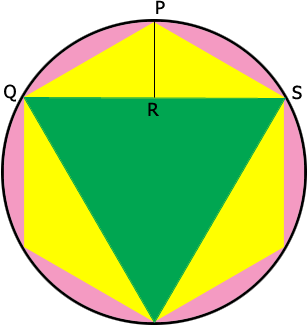SEARCH HOMEMath Central Quandaries & QueriesQuestion from Heidemarie, a student: The vertices of an equilateral triangle with side length of 10 sqrt 3 cm lie on a circle. Find the side length of the regular hexagon whose vertices lie on the same circle.Hi,$PR$ is perpendicular to $QS.$

What is the measure of the angle $SPQ?$

What is the measure of the angle $QSP?$

The result follows for knowing that in a "30, 60 90" triangle the ratio of the side lengths is $1:2:\sqrt{3}.$

PennyMath Central is supported by the University of Regina and The Pacific Institute for the Mathematical Sciences.